Tools, Technologies and Training for Healthcare Laboratories

# QC Selection Grid, Square 5

## MULTIRULE CHOICE: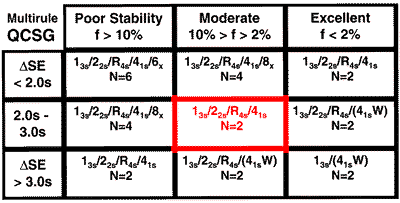You have a moderate number of problems and medically important errors are moderate in size (errors neither hard nor easy to detect). It is recommended that you increase the number of rules to four and apply the 41s rule across two runs (R=2) as a rejection rule. The power curves below show the rejection characteristics within one run (R=1) and across two runs (R=2):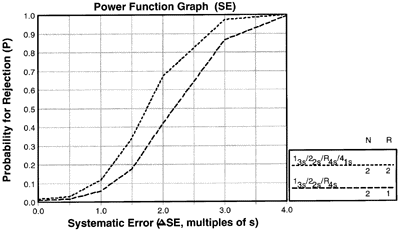Plot your critical-error on the x-axis of this power function graph. Draw a vertical line from that point to intersect the power curves. Where the curves and critical-error meet indicates how much error detection each control rule will provide.

# QC Selection Grid, Square 6

## MULTIRULE CHOICE: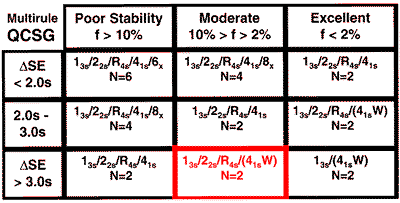You have a moderate number of problems, but the medically important errors are relatively large and therefore easy to detect. You should use a three rule multirule procedure with N=2 for within run detection and add a 41s rule to provide a more sensitive warning rule that works across two runs (R=2). The power curves below show the rejection characteristics within a run (R=1) and across two runs (R=2):Plot your critical-error on the x-axis of this power function graph. Draw a vertical line from that point to intersect the power curves. Where the curves and critical-error meet indicates how much error detection each control rule will provide.

# QC Selection Grid, Square 7

## MULTIRULE CHOICE: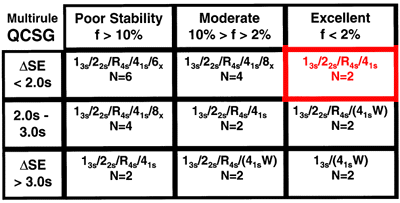You have few problems, but medically important errors are small and difficult to detect, therefore you need to increase the number of rules to four and apply the 41s rule across two runs (R=2) as a rejection rule. The power curves below show the rejection characteristics within one run (R=1) and across two runs (R=2):Plot your critical-error on the x-axis of this power function graph. Draw a vertical line from that point to intersect the power curves. Where the curves and critical-error meet indicates how much error detection each control rule will provide.

# MULTIRULE CHOICE: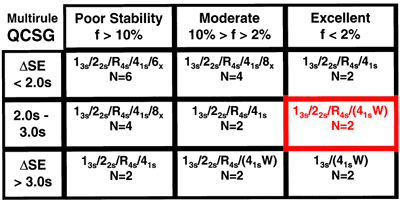You have few problems, but medically important errors are moderate in size, which means you should use at least three rules that can be applied to the two control measurements in a run (N=2) to improve error detection. You can also add a warning rule that works across two runs (R=2). The power curves below show the rejection characteristics within a run (R=1) and across two runs (R=2):Plot your critical-error on the x-axis of this power function graph. Draw a vertical line from that point to intersect the power curves. Where the curves and critical-error meet indicates how much error detection each control rule will provide.

# QC Selection Grid, square 9

Multirule Choice: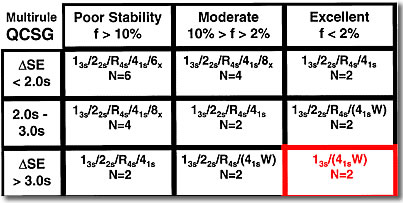Very cost-effective operation is possible because you have a very stable method and the medically important errors are large and easy to detect. You can actually use a single 13s rule with N=2 for rejection. If you want to look at data over two runs, you can add a 41s warning rule to alert analysts to prospectively check the method before the next run. The power curve below shows the rejection characteristics: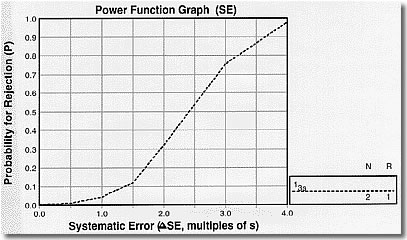Plot your critical-error on the x-axis of this power function graph. Draw a vertical line from that point to intersect the power curve. Where the curve and critical-error meet indicates how much error detection each control rule will provide.

Joomla SEF URLs by Artio